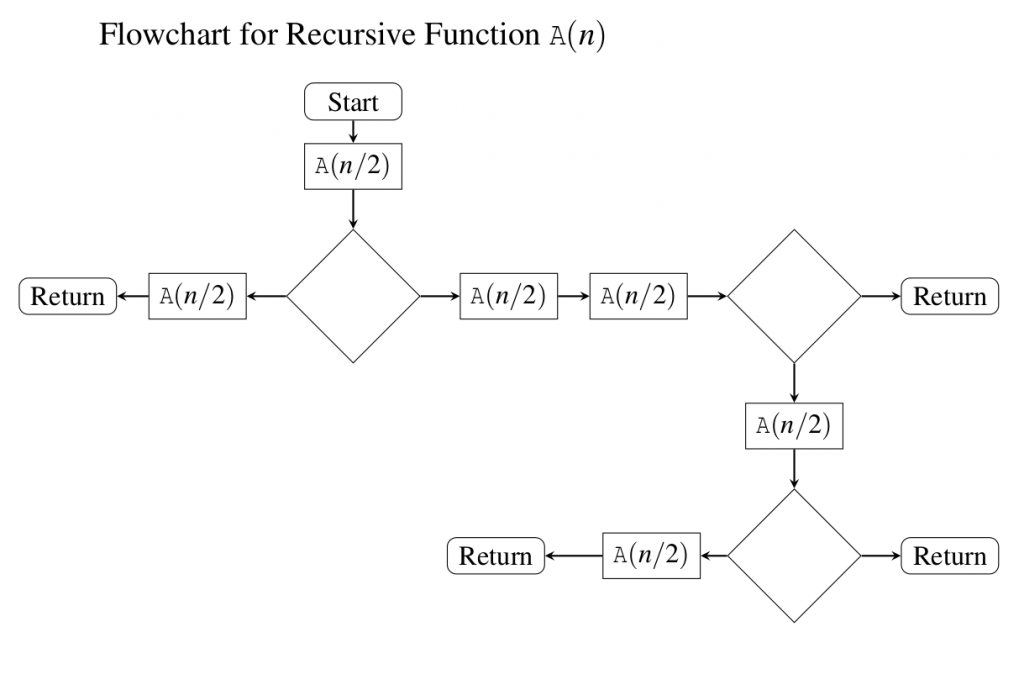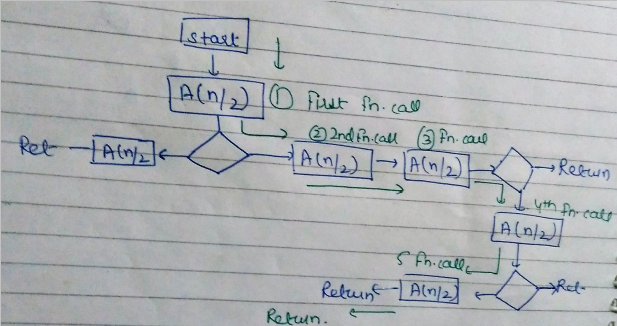# GATE | GATE-CS-2016 (Set 2) | Question 49

• Difficulty Level : Easy
• Last Updated : 28 Jun, 2021

The given diagram shows the flowchart for a recursive function A(n). Assume that all statements, except for the recursive calls, have O(1) time complexity. If the worst case time complexity of this function is O(nα), then the least possible value (accurate up to two decimal positions) of α is __________(A) 2.2 to 2.4
(B) 3.2 to 3.4
(C) 0 to 1.8
(D) 1

Explanation: The time complexity of a recurrence relation is the worst case time complexity. First we have to find out the number of function calls in worst case from the flow chart.
The worst case will be when all the conditions (diamond boxes) turn out towards non-returning paths taking the longest root. In the longest path we have 5 function calls.Attention reader! Don’t stop learning now.  Practice GATE exam well before the actual exam with the subject-wise and overall quizzes available in GATE Test Series Course.

Learn all GATE CS concepts with Free Live Classes on our youtube channel.

So the recurrence relation will be –
A(n) = 5A(n/2) + O(1) (O(1) because rest of the statements have O(1) complexity.)
Solving this recurrence relation using Master theorem –
a = 5 , b= 2 , f(n) =O(1) , nlogb a= nlog2 5 (case 1 master theorem)
A(n) =nlogb a
value of log 25 is 2.3219, so, the best option is option a

This explanation has been contributed by Parul Sharma.

Quiz of this Question

My Personal Notes arrow_drop_up